Next: Continuity equation for densities Up: Time-dependent density functional theory Previous: Time-dependent density functional theory

### Continuity equation for the scalar-isoscalar density

The CE now results from specifying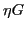to the local gauge transformation [13,Dobaczewski and Dudek(1995)] that is defined as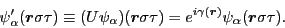(21)

Then, Eq. (13) gives: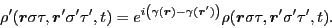(22)

Matrix elements of the local-gauge angle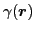are given by local integrals,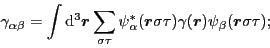(23)

therefore, from Eq. (13) again, the average value of the gauge angle,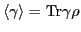, depends on the scalar-isoscalar local density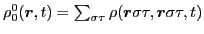, that is,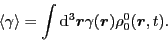(24)

Now, the assumed local-gauge invariance of the potential energy implies the equation of motion for the average value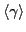, which from Eq. (20) reads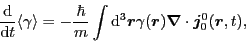(25)

where the standard scalar-isoscalar current is defined as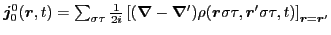.

We note here [13,Dobaczewski and Dudek(1995)], that the gauge invariance that corresponds to a specific dependence of the gauge angle on position,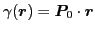, represents the Galilean invariance of the potential energy for the system boosted to momentum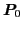. Then, equation of motion (25) simply represents the classical equation for the center-of-mass velocity,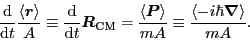(26)

In the general case, that is, when the potential energy is gauge-invariant and the gauge angleis an arbitrary function of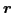, Eq. (25) gives the CE that reads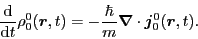(27)

Thus for a gauge-invariant potential energy density, the TDHF or TDDFT equation of motion implies the CE, that is, the gauge invariance is a sufficient condition for the validity of the CE. By proceeding in the opposite direction, we can prove that it is also a necessary condition. Indeed, the CE of Eq. (27) implies the first-order condition (19), and then the full gauge invariance stems from the fact that the gauge transformations form local U(1) groups.Next: Continuity equation for densities Up: Time-dependent density functional theory Previous: Time-dependent density functional theory
Jacek Dobaczewski 2011-11-11